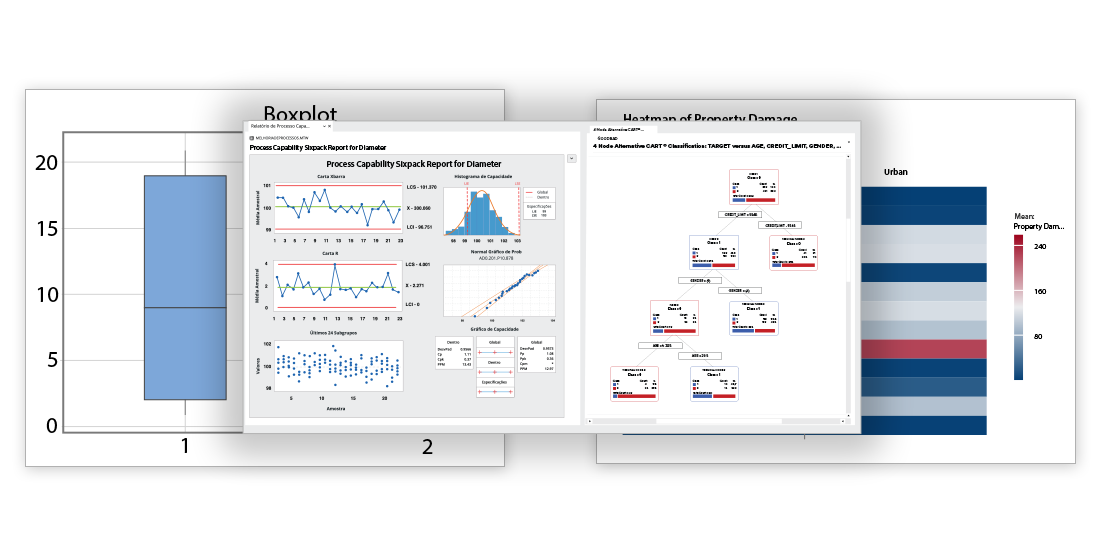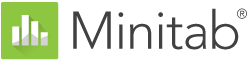# Features

MINITAB STATISTICAL SOFTWARE* New or Improved

### Assistant

• Measurement systems analysis
• Capability analysis
• Graphical analysis
• Hypothesis tests
• Regression
• DOE
• Control charts

### Graphics

• Graph Builder*
• Binned scatterplots, boxplots, bubble plots, bar charts, correlograms, dotplots, heatmaps, histograms, matrix plots, parallel plots, scatterplots, time series plots, etc.
• Contour and rotating 3D plots
• Probability and probability distribution plots
• Automatically update graphs as data change
• Brush graphs to explore points of interest
• Export: TIF, JPEG, PNG, BMP, GIF, EMF

### Basic Statistics

• Descriptive statistics
• One-sample Z-test, one- and two-sample t-tests, paired t-test
• One and two proportions tests
• One- and two-sample Poisson rate tests
• One and two variances tests
• Correlation and covariance
• Normality test
• Outlier test
• Poisson goodness-of-fit test

### Regression

• Cox regression
• Linear regression
• Nonlinear regression
• Binary, ordinal and nominal logistic regression
• Stability studies
• Partial least squares
• Orthogonal regression
• Poisson regression
• Plots: residual, factorial, contour, surface, etc.
• Stepwise: p-value, AICc, and BIC selection criterion
• Best subsets
• Response prediction and optimization
• Model validation

### Analysis of Variance

• ANOVA
• General linear models
• Mixed models
• MANOVA
• Multiple comparisons
• Response prediction and optimization
• Test for equal variances
• Plots: residual, factorial, contour, surface, etc.
• Analysis of means

### Measurement Systems Analysis

• Data collection worksheets
• Gage R&R Crossed
• Gage R&R Nested
• Gage R&R Expanded
• Gage run chart
• Gage linearity and bias
• Type 1 Gage Study
• Attribute Gage Study
• Attribute agreement analysis

### Quality Tools

• Run chart
• Pareto chart
• Cause-and-effect diagram
• Variables control charts: XBar, R, S, XBar-R, XBar-S, I, MR, I-MR, I-MR-R/S, zone, Z-MR
• Attributes control charts: P, NP, C, U, Laney P’ and U’
• Time-weighted control charts: MA, EWMA, CUSUM
• Multivariate control charts: T2, generalized variance, MEWMA
• Rare events charts: G and T
• Historical/shift-in-process charts
• Box-Cox and Johnson transformations
• Individual distribution identification
• Process capability: normal, non-normal, attribute, batch
• Process Capability Sixpack™
• Tolerance intervals
• Acceptance sampling and OC curves
• Multi-Vari chart
• Variability chart

### Design of Experiments

• Definitive screening designs
• Plackett-Burman designs
• Two-level factorial designs
• Split-plot designs
• General factorial designs
• Response surface designs
• Mixture designs
• D-optimal and distance-based designs
• Taguchi designs
• User-specified designs
• Analyze binary responses
• Analyze variability for factorial designs
• Botched runs
• Effects plots: normal, half-normal, Pareto
• Response prediction and optimization
• Plots: residual, main effects, interaction, cube, contour, surface, wireframe

### Reliability/Survival

• Parametric and nonparametric distribution analysis
• Goodness-of-fit measures
• Exact failure, right-, left-, and interval-censored data
• Accelerated life testing
• Regression with life data
• Test plans
• Threshold parameter distributions
• Repairable systems
• Multiple failure modes
• Probit analysis
• Weibayes analysis
• Plots: distribution, probability, hazard, survival
• Warranty analysis

### Power and Sample Size

• Sample size for estimation
• Sample size for tolerance intervals
• One-sample Z, one- and two-sample t
• Paired t
• One and two proportions
• One- and two-sample Poisson rates
• One and two variances
• Equivalence tests
• One-Way ANOVA
• Two-level, Plackett-Burman and general full factorial designs
• Power curves

### Predictive Analytics

• Automated Machine Learning
• CART® Classification
• CART® Regression
• MARS®
• Random Forests® Classification
• Random Forests® Regression
• TreeNet® Classification
• TreeNet® Regression

### Multivariate

• Principal components analysis
• Factor analysis
• Discriminant analysis
• Cluster analysis
• Correspondence analysis
• Item analysis and Cronbach’s alpha

### Time Series and Forecasting

• Time series plots
• Trend analysis
• Decomposition
• Moving average
• Exponential smoothing
• Winters’ method
• Auto-, partial auto-, and cross correlation functions
• ARIMA
• Box-Cox Transformation*
• Augmented Dickey-Fuller Test*
• Forecast with Best ARIMA Model*

### Nonparametrics

• Sign test
• Wilcoxon test
• Mann-Whitney test
• Kruskal-Wallis test
• Mood’s median test
• Friedman test
• Runs test

### Equivalence Tests

• One- and two-sample, paired 2x2 crossover design

### Tables

• Chi-square, Fisher’s exact, and other tests
• Chi-square goodness-of-fit test
• Tally and cross tabulation

### Simulations and Distributions

• Random number generator
• Probability density, cumulative distribution, and inverse cumulative distribution functions
• Random sampling
• Bootstrapping and randomization tests